# OCTOBER 2019

## Gratitude: IIT Community Expands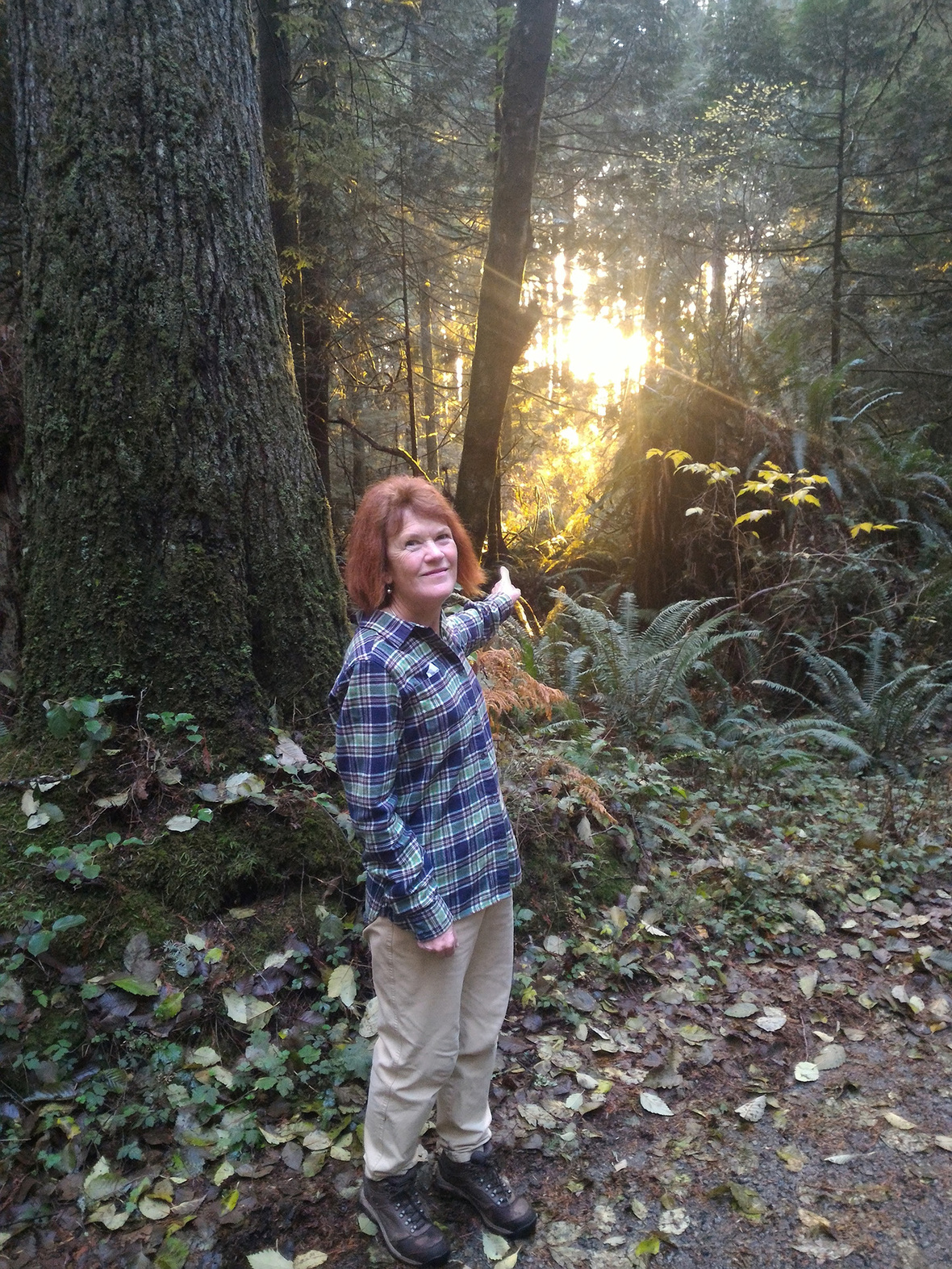Dear Friend,

I am happy to announce another new IIT co-creation project is complete: our website AND our first self-study e-course Transform Yourself are now available in Spanish! A very special thank you to all of our IIT co-creators who are expanding the IIT community around the world.

Our self-study e-courses special launch price is on until October 30. If you don't have all of our courses yet, now is the time!

 table div table+table+table+table+table div table{width:100%;padding:0}table div table+table+table+table+table div table img{width:96.23%;padding:0;float:none}table div table+table+table+table+table div table td{width:100%;padding:0 1.88% 18px}/* styles *//* styles */ "We count by changes and events within us. Not by years." --Charles Dickens
 table div table+table+table+table+table+table+table div table{width:100%;padding:0}table div table+table+table+table+table+table+table div table img{width:96.23%;padding:0;float:none}table div table+table+table+table+table+table+table div table td{width:100%;padding:0 1.88% 18px}/* styles */## IIT Website & Transform Yourself e-course now in Spanish!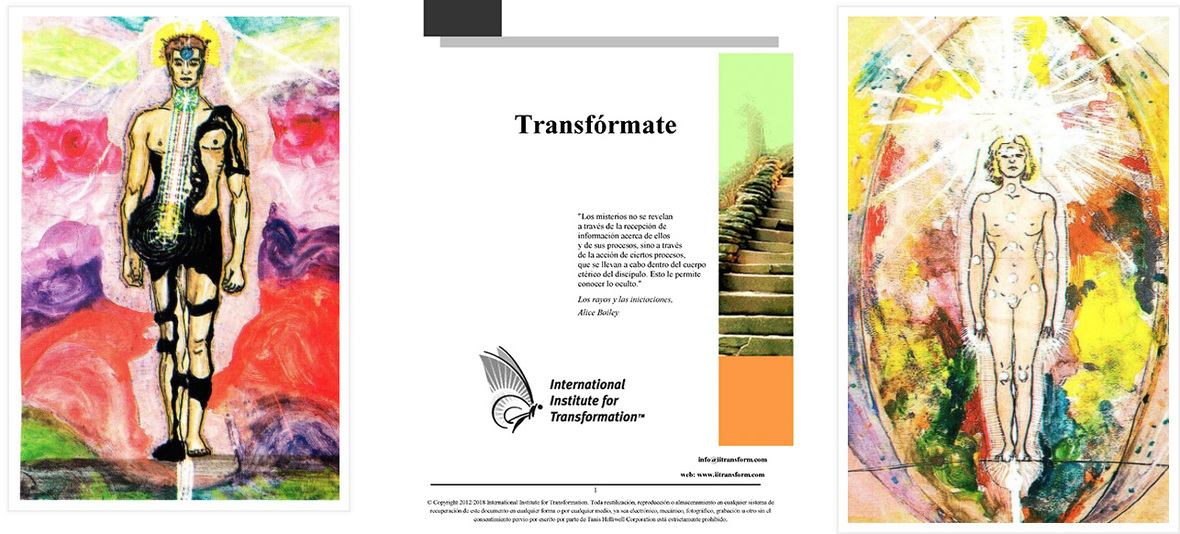/* styles */ Back in February, one of my dear readers, Ornella Quinteros, contacted me from her home in Argentina to tell me how much she enjoyed my classic book, Summer With the Leprechauns. Little did either of us know that we would be co-creating so much together! Ornella told me that she is an English-Spanish translator, and the idea took hold. Almost 9 months later, our new creations were born: IIT's first and most popular course, Transform Yourself, is now also available for study in Spanish. View and order your copy of the new Transfórmate (just click on the Spanish flag for the translated page and link). Special price: only \$127 until Oct. 30! Our IIT website is now available in Spanish - just click on the English/Spanish flag icon at the bottom of your screen to view it here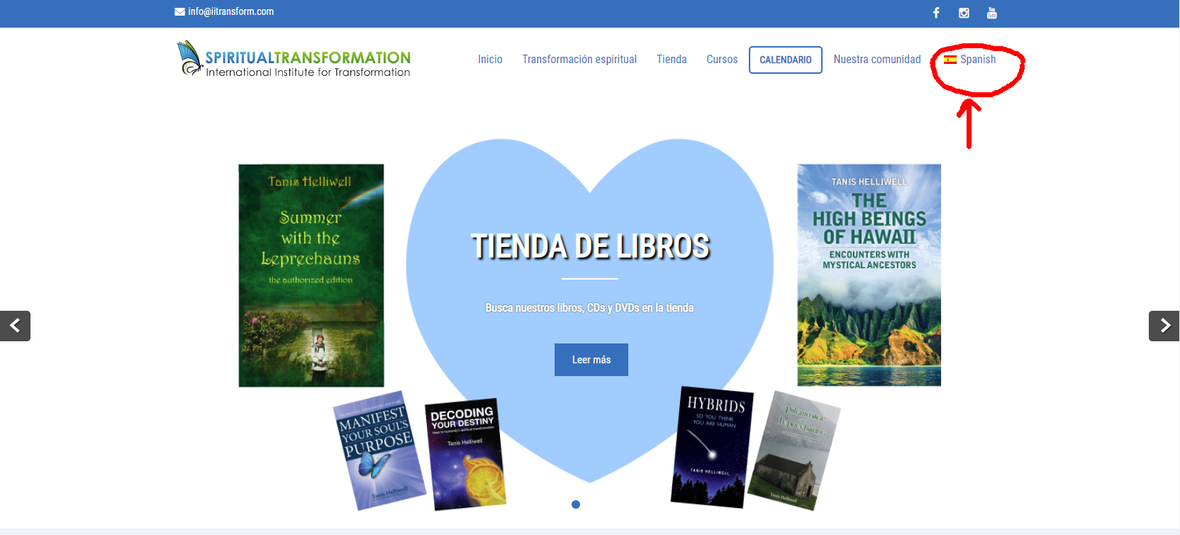table div table+table+table+table+table+table+table+table+table+table+table+table+table div table{width:100%;padding:0}table div table+table+table+table+table+table+table+table+table+table+table+table+table div table img{width:96.23%;padding:0;float:none}table div table+table+table+table+table+table+table+table+table+table+table+table+table div table td{width:100%;padding:0 1.88% 18px}/* styles */# Some of the Ways We Practice Co-creation at IIT

Our newsletters and websites are translated by volunteers who speak non-English languages and who practice world service in offering their time and gifts for us to reach more people in more countries.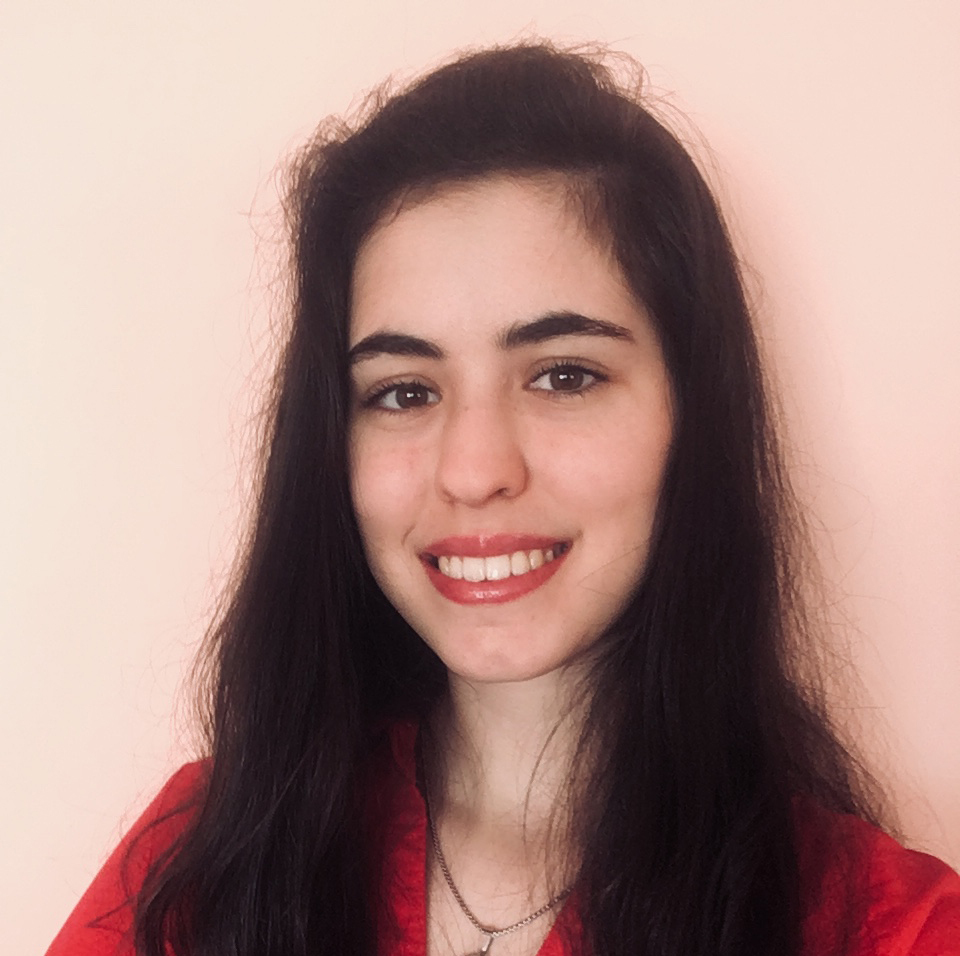Ornella Quinteros, Spanish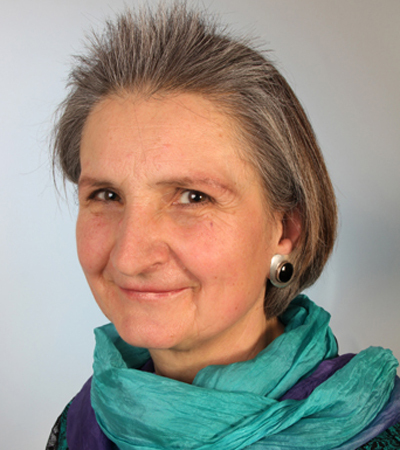Monika Bernegg, German
 /* styles */ All of our e-courses are co-created based on Tanis Helliwell’s courses and books. Sometimes two people work together and sometimes four depending on the skills, physical ability, and willingness. Our newest course, Ancestor & Family Healing, was co-created in German by Verena Mezger, Irene Ocklenburg, Monika Bernegg and Viola Ehlers. This newest IIT self-study e-course is launching today in German!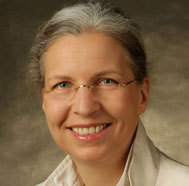Verena Mezger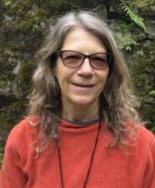Irene OcklenburgMonika Bernegg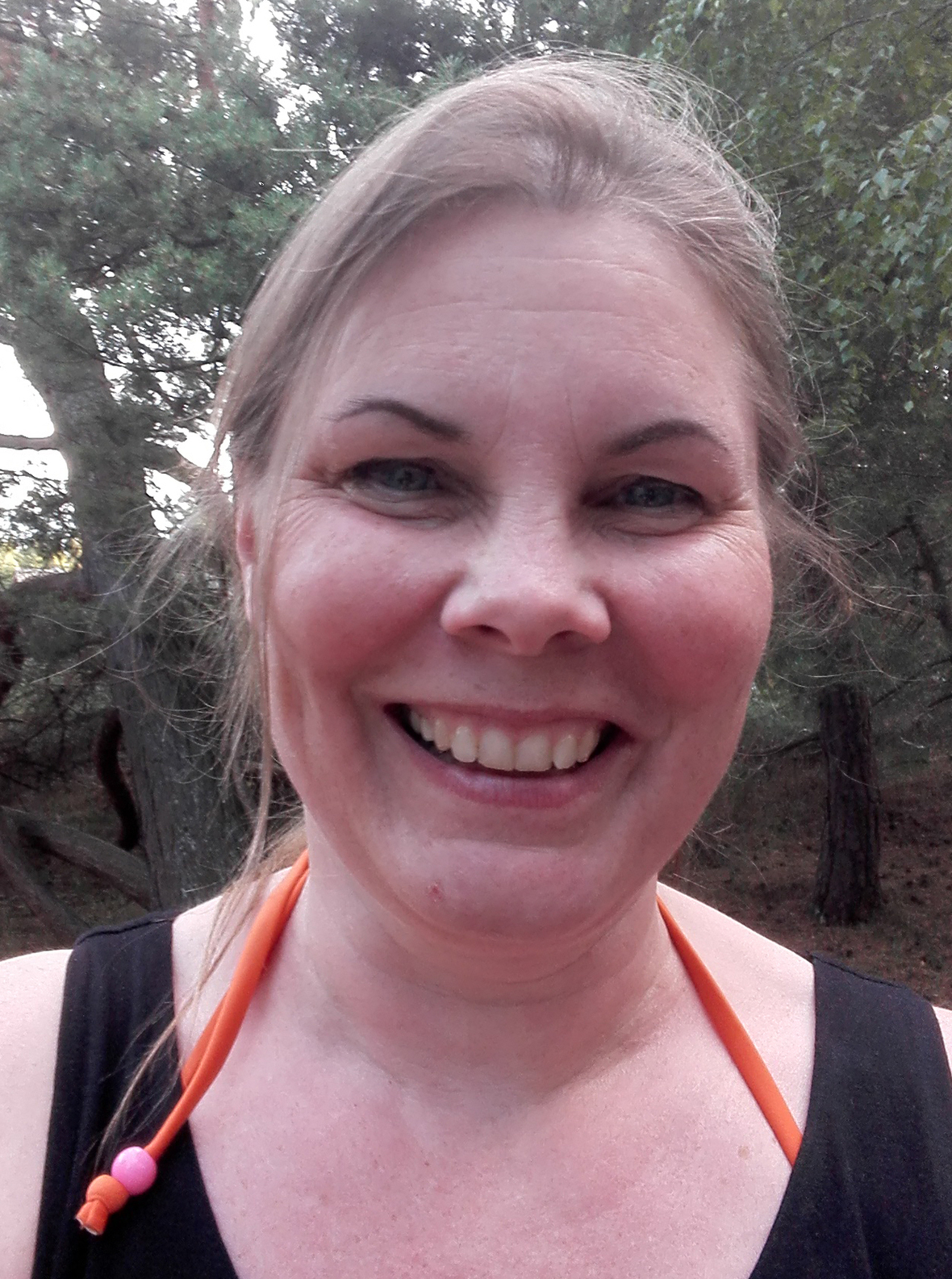Viola Ehlers
 /* styles */ E-courses are also in the process of being created in both Spanish and Bulgarian. Our Spanish version of Transform Yourself - Transfórmate - is launching today!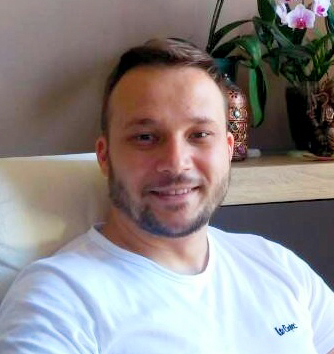Todor Simeonov, BulgarianOrnella Quinteros, Spanish
 table div table+table+table+table+table+table+table+table+table+table+table+table+table+table+table+table+table+table+table+table+table+table div table{width:100%;padding:0}table div table+table+table+table+table+table+table+table+table+table+table+table+table+table+table+table+table+table+table+table+table+table div table img{width:96.23%;padding:0;float:none}table div table+table+table+table+table+table+table+table+table+table+table+table+table+table+table+table+table+table+table+table+table+table div table td{width:100%;padding:0 1.88% 18px}/* styles */## All e-courses only \$127 each until Oct. 30!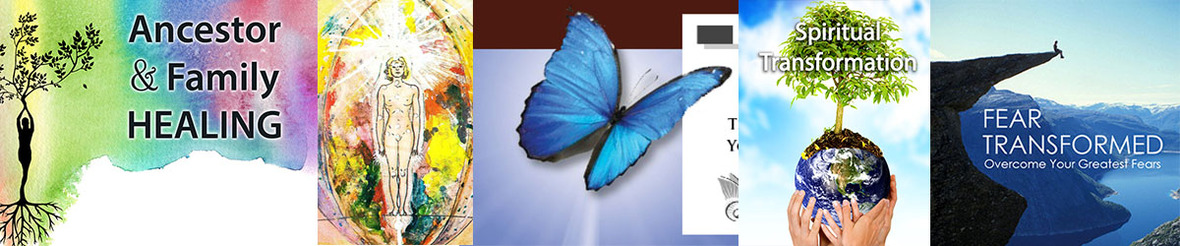## Regular price \$147

Our five e-courses can be taken either individually or in groups that are led by dedicated people around the world. Our e-course hosts wish to help others in their spiritual transformation, as well as being helped by others in a group setting. All of our e-courses are available in both English and German:

1 NEW: Ancestor & Family Healing - learn how your ancestors influence your life today and how you can contact them for healing. (Launching TODAY in German!)

2 Transform Yourself - let go of old patterns that no longer serve you, and unite your personality with your soul’s purpose. (Launching today in Spanish! Also available in Italian.)

3 Transform Your Work - improve your relationships, have a happier work environment and have greater peace and joy.

4 Spiritual Transformation - Are you feeling restless or dissatisfied? Are blame and shame ruling your inner and outer world? You may be going through a spiritual transformation.

5 Fear Transformed - Is fear controlling your life? Recognize the signs and get the guidance you need along the journey.

 table div table+table+table+table+table+table+table+table+table+table+table+table+table+table+table+table+table+table+table+table+table+table+table+table+table+table+table div table{width:100%;padding:0}table div table+table+table+table+table+table+table+table+table+table+table+table+table+table+table+table+table+table+table+table+table+table+table+table+table+table+table div table img{width:96.23%;padding:0;float:none}table div table+table+table+table+table+table+table+table+table+table+table+table+table+table+table+table+table+table+table+table+table+table+table+table+table+table+table div table td{width:100%;padding:0 1.88% 18px}/* styles */## Join my YouTube Q&A session on Ancestor & Family Healing

 table.module-29{width:99.81%;padding:0}table div table+table+table+table+table+table+table+table+table+table+table+table+table+table+table+table+table+table+table+table+table+table+table+table+table+table+table+table+table+table div table{width:99.81%;float:none;margin-left:auto;margin-right:auto;padding:0}table div table+table+table+table+table+table+table+table+table+table+table+table+table+table+table+table+table+table+table+table+table+table+table+table+table+table+table+table+table+table div table a{border:0 none;text-decoration:none}table div table+table+table+table+table+table+table+table+table+table+table+table+table+table+table+table+table+table+table+table+table+table+table+table+table+table+table+table+table+table div table img{width:100%!important;border:0 none;text-decoration:none}table div table+table+table+table+table+table+table+table+table+table+table+table+table+table+table+table+table+table+table+table+table+table+table+table+table+table+table+table+table+table div table td{width:100%;padding:0}/* styles */
 table div table+table+table+table+table+table+table+table+table+table+table+table+table+table+table+table+table+table+table+table+table+table+table+table+table+table+table+table+table+table+table+table div table{width:100%;padding:0}table div table+table+table+table+table+table+table+table+table+table+table+table+table+table+table+table+table+table+table+table+table+table+table+table+table+table+table+table+table+table+table+table div table img{width:96.23%;padding:0;float:none}table div table+table+table+table+table+table+table+table+table+table+table+table+table+table+table+table+table+table+table+table+table+table+table+table+table+table+table+table+table+table+table+table div table td{width:100%;padding:0 1.88% 18px}/* styles */## ICELAND 2020: A magical mystery tour

 /* styles */ I am very happy to share with you that we are planning a mystical tour of Iceland for May 2020. If you're interested, please let us know so that we can get a good idea of numbers and plan accordingly. Email us at info(at)iitransform.com. More information coming soon! Check our schedule HERE for updates.
 table div table+table+table+table+table+table+table+table+table+table+table+table+table+table+table+table+table+table+table+table+table+table+table+table+table+table+table+table+table+table+table+table+table+table+table div table{width:100%;padding:0}table div table+table+table+table+table+table+table+table+table+table+table+table+table+table+table+table+table+table+table+table+table+table+table+table+table+table+table+table+table+table+table+table+table+table+table div table img{width:96.23%;padding:0;float:none}table div table+table+table+table+table+table+table+table+table+table+table+table+table+table+table+table+table+table+table+table+table+table+table+table+table+table+table+table+table+table+table+table+table+table+table div table td{width:100%;padding:0 1.88% 18px}/* styles */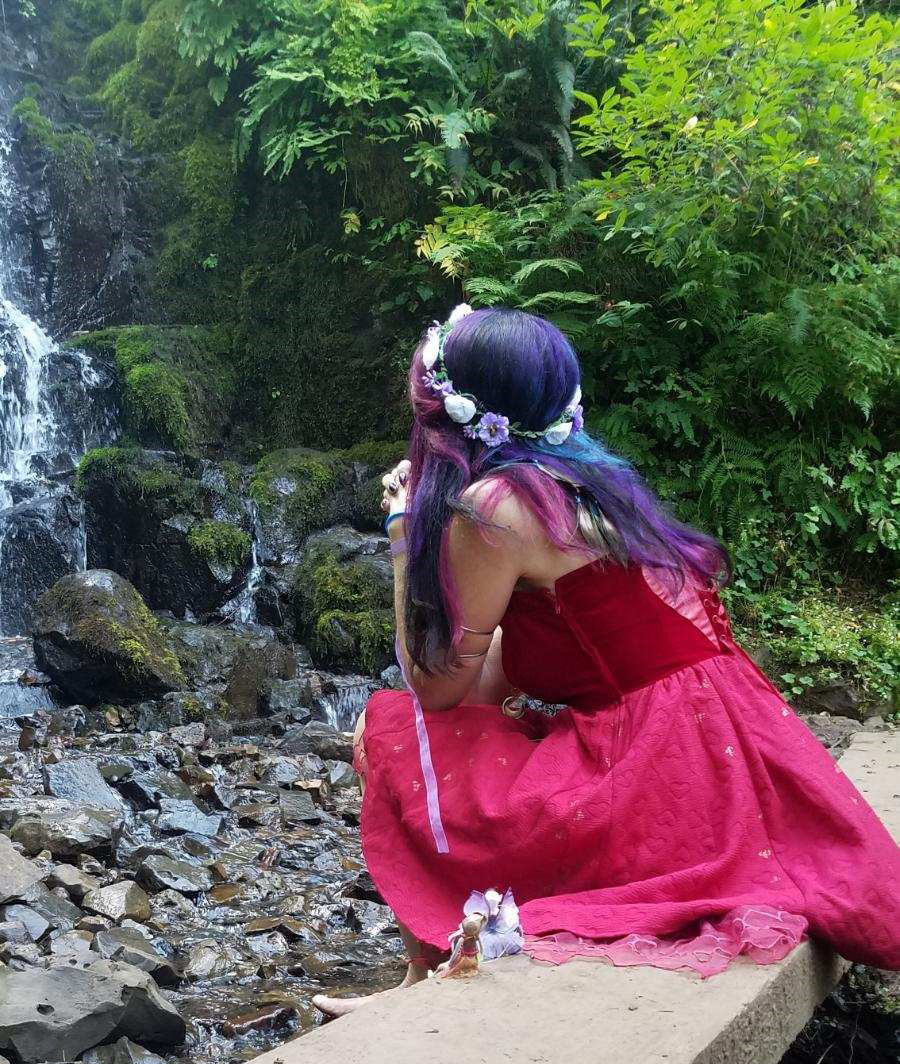Lara and her fairy friend.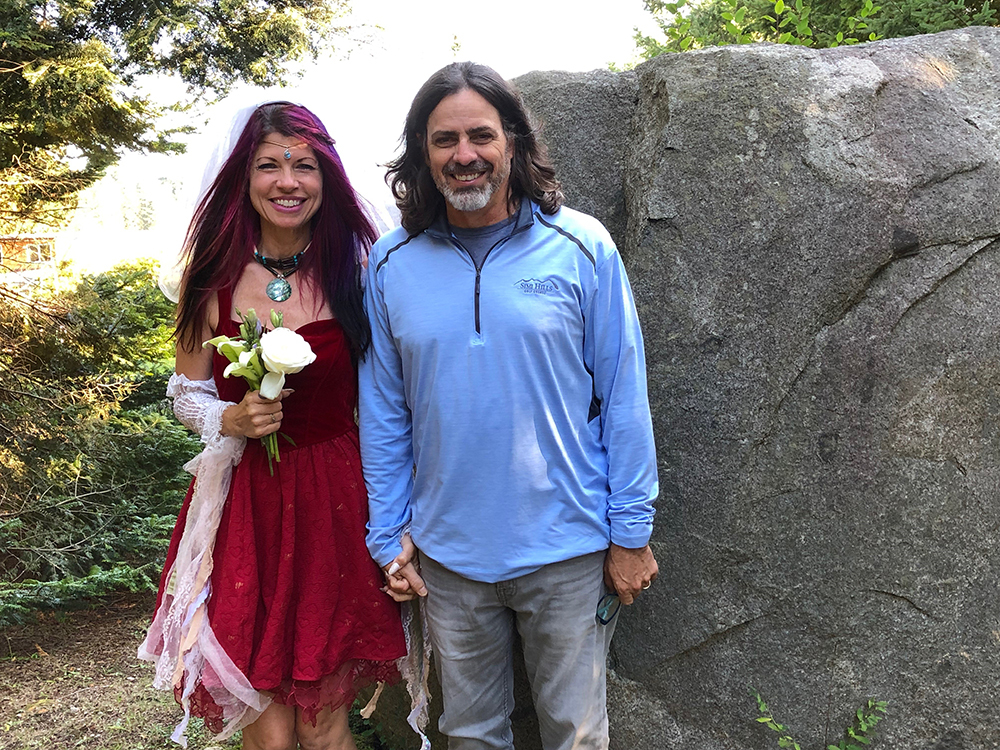Faerie wedding: Lara & Dan at Diamond Heart.
 table div table+table+table+table+table+table+table+table+table+table+table+table+table+table+table+table+table+table+table+table+table+table+table+table+table+table+table+table+table+table+table+table+table+table+table+table+table+table+table+table div table{width:100%;padding:0}table div table+table+table+table+table+table+table+table+table+table+table+table+table+table+table+table+table+table+table+table+table+table+table+table+table+table+table+table+table+table+table+table+table+table+table+table+table+table+table+table div table img{width:96.23%;padding:0;float:none}table div table+table+table+table+table+table+table+table+table+table+table+table+table+table+table+table+table+table+table+table+table+table+table+table+table+table+table+table+table+table+table+table+table+table+table+table+table+table+table+table div table td{width:100%;padding:0 1.88% 18px}/* styles */